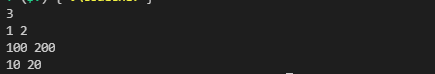Every problem starts with a Problem Statement. It tells you in detail about the task to be solved. Usually, although not necessarily, it is accompanied by a story. As a competitive programmer, it is your job to break the problem statement and figure out exactly what it is asking.

Shivam is the youngest programmer in the world, he is just 12 years old. Shivam is learning programming and today he is writing his first program.

The task is very simple: given two integers A and B, write a program to add these two numbers and output it.

Input

This section tells you the format in which your program should receive the input.

The first line contains an integer T, the total number of test cases. Then follow T lines, each line contains two integers A and B.

Output

This section tells us the format in which your program should give the output

For each test case, add A and B and display the sum in a new line.

Take special care for the output format; everything your program prints is considered “output”, so if you output some debugging statements like “Please enter T” or print something like: “The answer is: ”, this will be considered as part of your answer, and because it does not satisfy the output format, it will be marked wrong, even if your answer is otherwise correct!

Constraints

This section tells you the maximum and minimum possible values the variables in the problem statement can take. You do not need to check these constraints in your program. You can safely assume that the input given to your program will be in the given range of constraints.

1 ≤ T ≤ 1000

0 ≤ A, B ≤ 10000

Sample Input 1

3

3 8

25 50

10 40

Sample output:

11

75

50codechef problem solution in cpp

#include <iostream>

using namespace std;

int main(){
int length;
int a, b;
cin>>length;
int *t = new int[length];
for (int i = 0; i < length; i++ )
{
cin>>a>>b; cin.ignore();
t[i] = a+b;
}
for (int i = 0; i < length; i++){
cout << t[i] << endl;
}
return 0;
}